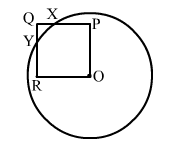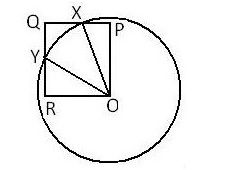# In the adjoining figure, OPQR is a square. A circle drawn with centre O cuts the square at X and Y.

Question:

In the adjoining figure, OPQR is a square. A circle drawn with centre O cuts the square at X and Y. Prove that QX = QY.Solution:

Given: OPQR is a square. A circle with centre O cuts the square at X and Y.
To prove: QX = QY
Construction: Join OX and OY.Proof:
In ΔOXP and ΔOYR, we have:
∠OPX = ∠ORY      (90° each)
OX = OY                (Radii of a circle)
OP = OR                (Sides of a square)
∴ ΔOXP ≅ ΔOYR    (BY RHS congruency rule)
PX = RY              (By CPCT)
⇒ PQ - PX = QR - RY   (PQ and QR are sides of a square)
⇒ QX = QY
Hence, proved.